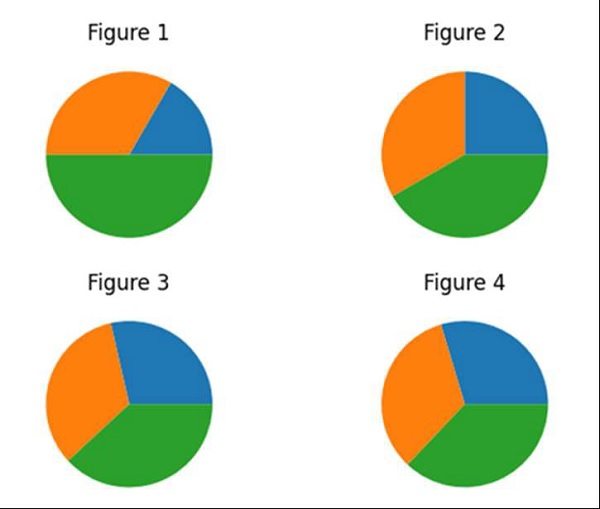# How to display multiple images in one figure correctly in matplotlib?

To display multiple images in one figure, we can follow the steps given below −

• Initialize the number of rows and cols. nrows*ncols subplot will be created in the current figure. nrows = 2 and ncols = 2, i.e., 2*2 = 4 subplots can be created.

• Now add the figures at different indices from 1 to 4.

• Use plt.subplot(2, 2, 1) to add new images, i.e., pie at index 1.

• To plot a pie chart, pass a list of numbers. Pie charts will be split into the size of list and %age section will depend upon the values in the list.

• Set the title of the subplot, i.e., “Figure 1”.

• Use plt.subplot(2, 2, 2) to add new images, i.e., pie at index 2.

• Use plt.pie() to create a new pie chart.

• Set the title of the subplot, i.e., “Figure 2”.

• Use plt.subplot(2, 2, 3) to add new images i.e., pie at index 3.

• Use plt.pie() to create a new pie chart.

• Set the title of the subplot i.e., “Figure 3”.

• Use plt.subplot(2, 2, 4) to add new images i.e., pie at index 4.

• Use plt.pie() to create a new pie chart.

• Set the title of the subplot i.e., “Figure 4”.

• Use plt.show(), to show the figure.

## Example

import matplotlib.pyplot as plt

rows, cols = 2, 2
plt.subplot(rows, cols, 1)
plt.pie([1, 2, 3])
plt.title("Figure 1")
plt.subplot(rows, cols, 2)
plt.pie([3, 4, 5])
plt.title("Figure 2")
plt.subplot(rows, cols, 3)
plt.pie([6, 7, 8])
plt.title("Figure 3")
plt.subplot(rows, cols, 4)
plt.pie([8, 9, 10])
plt.title("Figure 4")

plt.show()

## Output